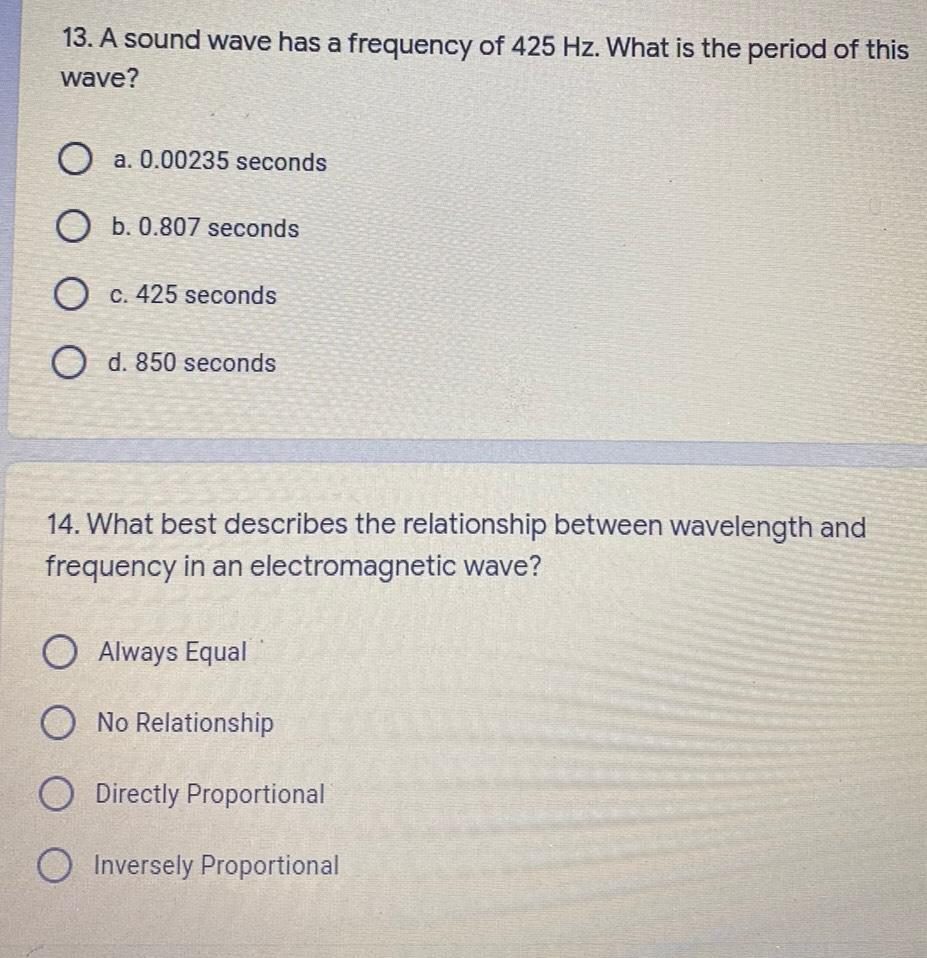Question:

# 13. A sound wave has a frequency of 425 Hz. What is the period of this wave? O a. 0.00235 seconds O b. 0.807 seconds . O c. 42513. A sound wave has a frequency of 425 Hz. What is the period of this wave? O a. 0.00235 seconds O b. 0.807 seconds . O c. 425 seconds O d. 850 seconds 14. What best describes the relationship between wavelength and frequency in an electromagnetic wave? O Always Equal O No Relationship O Directly Proportional Inversely Proportional